# JP2004252831A - Statistical delay simulation device for lsi and its simulation method - Google Patents

Statistical delay simulation device for lsi and its simulation method Download PDF

## Info

Publication number
JP2004252831A
JP2004252831A JP2003044029A JP2003044029A JP2004252831A JP 2004252831 A JP2004252831 A JP 2004252831A JP 2003044029 A JP2003044029 A JP 2003044029A JP 2003044029 A JP2003044029 A JP 2003044029A JP 2004252831 A JP2004252831 A JP 2004252831A
Authority
JP
Japan
Prior art keywords
delay
lsi
statistical
variation
circuit
Prior art date
Legal status (The legal status is an assumption and is not a legal conclusion. Google has not performed a legal analysis and makes no representation as to the accuracy of the status listed.)
Pending
Application number
JP2003044029A
Other languages
Japanese (ja)
Inventor
Hirokazu Yonezawa

Original Assignee
Matsushita Electric Ind Co Ltd

Priority date (The priority date is an assumption and is not a legal conclusion. Google has not performed a legal analysis and makes no representation as to the accuracy of the date listed.)
Filing date
Publication date
Application filed by Matsushita Electric Ind Co Ltd, 松下電器産業株式会社 filed Critical Matsushita Electric Ind Co Ltd
Priority to JP2003044029A priority Critical patent/JP2004252831A/en
Publication of JP2004252831A publication Critical patent/JP2004252831A/en
Application status is Pending legal-status Critical

• 238000000034 methods Methods 0 abstract 1

## Images

•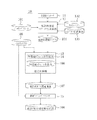•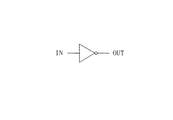•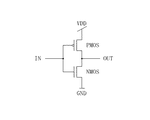•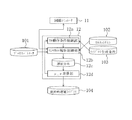•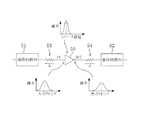•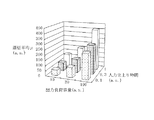•••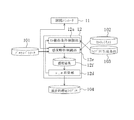•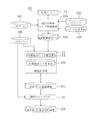•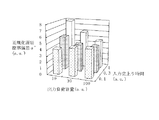•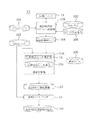•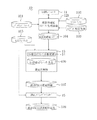•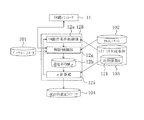••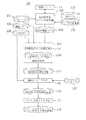•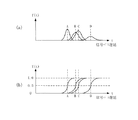•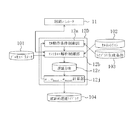•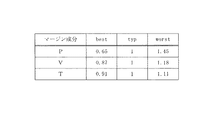•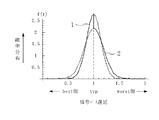••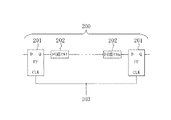•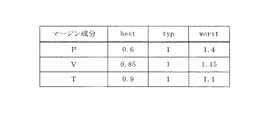## Classifications

• GPHYSICS
• G06COMPUTING; CALCULATING; COUNTING
• G06FELECTRIC DIGITAL DATA PROCESSING
• G06F30/30Circuit design
• G06F30/32Circuit design at the digital level
• G06F30/33Design verification, e.g. functional simulation or model checking
• GPHYSICS
• G06COMPUTING; CALCULATING; COUNTING
• G06FELECTRIC DIGITAL DATA PROCESSING
• G06F30/30Circuit design
• G06F30/36Circuit design at the analogue level
• G06F30/367Design verification, e.g. using simulation, simulation program with integrated circuit emphasis [SPICE], direct methods or relaxation methods
• GPHYSICS
• G06COMPUTING; CALCULATING; COUNTING
• G06FELECTRIC DIGITAL DATA PROCESSING
• G06F2111/00Details relating to CAD techniques

## Abstract

<P>PROBLEM TO BE SOLVED: To estimate dispersion of a delay in an LSI in a design step for easily and surely reflecting the estimation result to delay simulation of the LSI. <P>SOLUTION: A statistical delay simulation device 10 is provided with a circuit simulator 11 simulating circuit operation of a circuit cell constituting the LSI, a statistical delay library creation device 12 creating a statistical delay library 104 describing dependency of delay dispersion in each circuit on a predetermined operation condition according to a process parameter 101 and the like, a delay calculator 13 calculating a delay quantity of each circuit cell and creating a statistical LSI delay information file 107 including the delay data, and a static timing analyzer 15 simulating operations including the delay dispersion of the LSI on the basis of the data of the statistical LSI delay information file 107 and creating a statistical LSI delay analysis result file 108. <P>COPYRIGHT: (C)2004,JPO&NCIPI

## Description


TECHNICAL FIELD OF THE INVENTION
The present invention relates to a statistical delay simulation apparatus and a simulation method for estimating a variation in delay of an LSI due to a manufacturing variation factor and reflecting the result in a delay simulation of the LSI.

[Prior art]
Since the miniaturization of transistors and the degree of integration are rapidly improving with the development of manufacturing technology, CMIS semiconductor integrated circuit devices (hereinafter abbreviated as LSI) have recently been required to realize various functions on one chip. Has reached.

Incidentally, an LSI is usually developed with a design margin, that is, a design margin at the time of manufacture. There are various factors to consider the design margin.For example, the electrical characteristics of transistors constituting a circuit vary due to manufacturing reasons, and the electrical characteristics of the circuit also vary due to a change in the operating environment voltage or temperature of the circuit. Design margins must usually be considered due to variations in characteristics.

As shown in FIG. 22, in general, an LSI can be decomposed into a signal path 200 including n stages (where n is an integer of 1 or more) of circuit cells 202 between flip-flops 201.

The circuit cell 202 generally includes a logic circuit such as an inverter, a NAND, or a NOR, and these logic circuits are connected to each other by wiring. At this time, a delay generated when the signal propagates through a series of circuit cells 202 included in the signal path 200 is caused by a cycle time of the clock signal 203 (usually, an operating frequency or a reciprocal of the clock frequency, or a cycle of an integral multiple thereof). Is required to be designed to fall within a predetermined period determined by This relationship is shown in equation (1).

t cycle ≧ Σt i + K (where i = 1, 2,..., N is an integer) (1)
Where t cycle Is the cycle time which is the design target characteristic, Δt i Is the signal propagation delay t between the input and output terminals of the circuit cell i located between the flip-flops 201 i Is the sum of the setup time of the flip-flop 201 and the skew of the clock signal 203.

Originally, the design margin is expressed by converting various delay fluctuation factors called derating factors into coefficients. For example, as shown in the following equation (2), a power-saving design is performed by simply estimating a worst-case delay value from a standard (typical) delay value. Where t worst Is the worst value of each signal path delay, t type Is a standard value of each signal path delay, P is a delay variation coefficient due to manufacturing variation, V is a delay variation coefficient due to a power supply voltage width, and T is a delay variation coefficient due to a temperature width. In this case, the worst value of the design margin is simply obtained by first obtaining the standard value of the entire signal path delay of the LSI and multiplying the standard value by the derating factors P, V, and T, respectively. Here, the right side in the equation (2) is Δt in the equation (1). i Is equivalent to

FIG. 23 shows an example of the derating factor. When these are applied to equation (2), the worst delay value t worst Is the standard (type) delay value t type Is obtained by equation (3) using Also, from equation (4), the best (best) delay value t best Can be obtained in the same manner, and the operation of the LSI under the conditions of best and worst can be confirmed at the time of design.

t worst = T type × P × V × T (2)
t worst = T type × 1.4 × 1.15 × 1.1 (3)
t best = T type × 0.6 × 0.85 × 0.9 (4)
There is a trade-off between the quality and the performance of the LSI, and setting an excessive margin of the LSI is safe, but increases the design, but decreases the performance (eg, operating frequency) of the LSI.

On the other hand, if the margin is too small, there is a risk that the quality is insufficient and a malfunction occurs. Therefore, unless a design environment capable of evaluating an appropriate margin with no excess or deficiency and performing a design based on the margin is not prepared, an LSI having the same performance and quality as a recent digital signal processor is efficiently developed. It becomes difficult.

In a method of providing a design margin by delay calculation performed by applying a fixed derating factor to all signal paths of an LSI, such as using the worst condition shown in FIG. 23, an optimum margin for each signal path is evaluated. And cannot be set, so that it is easy to fall into an excessive margin as a whole.

Therefore, a method of statistically calculating the delay of an LSI without using a derating factor is known (see Patent Document 1). This is because a variation in delay of circuit cells included in an LSI, that is, a probability distribution of delay is represented by a histogram, and a probability distribution of delay for each circuit cell along a signal path in the LSI is calculated by an operation between the obtained histograms. This is a method in which the reliability of the design is evaluated based on the variation (probability distribution) of the determined output delay of the signal path. According to this method, the variation in delay according to the signal path can be individually evaluated, so that the design margin can be designed without excess or deficiency as compared with the case where the derating factor is used.

[Patent Document 1]
U.S. Pat. No. 5,383,167

[Problems to be solved by the invention]
However, in the conventional LSI statistical delay simulation method disclosed in Patent Document 2, since the delay variation of circuit cells is expressed using a histogram, it is possible to give an arbitrary probability distribution (shape). In addition, there is a problem that the variation in the delay of the signal path must be calculated as a number of convolutions using a histogram between the probability distributions, and the calculation becomes complicated.

In addition, since the variation in the delay of the circuit cell is a fixed value for each circuit cell, and the change due to the connection state and the arrangement state of each circuit cell in the LSI is not taken into consideration, the reliability of the finally obtained operation result is low. There is a problem of low.

Further, in the initial stage of LSI development, there is a demand to grasp the range of the upper and lower limits of the delay variation even in the outline. On the other hand, the conventional method has a problem that the range of the upper and lower limits of the delay variation cannot be easily grasped.

In addition, the conventional method assumes that the delay variation has a symmetric distribution on the best side and the worst side with respect to the type value as shown in FIG. Actually, there is a case where it is asymmetrical around the type value, and the fluctuation amount on the best side or the worst side is also different. However, this asymmetric phenomenon can be easily handled other than by the complicated calculation using the histogram. Can not.

The present invention has been made in view of the above-described conventional problems, and has as its object to estimate a variation in delay in an LSI at a design stage and to easily and surely reflect the estimation result in a delay simulation of the LSI. .

[Means for Solving the Problems]
In order to achieve the above object, an LSI statistical delay simulation apparatus according to the present invention is directed to a simulation apparatus that analyzes a statistical delay of an LSI including a plurality of circuit cells in a design stage, and a characteristic of each circuit cell is analyzed. Cell delay variation estimating means for estimating the variation in delay of each circuit cell based on the value of the operating condition for each circuit cell during the operation of the LSI, with reference to a statistical characteristic library representing the dependence of the variation on the operating condition And simulates an operation including a variation in the delay of the LSI estimated by the cell delay variation estimating means.

According to the LSI statistical delay simulation apparatus of the present invention, the apparatus includes the cell delay variation estimating means for estimating the delay variation of each circuit cell based on the value of the operating condition for each circuit cell during the operation of the LSI. The delay variation of the circuit cell can be obtained in consideration of the operating conditions in the circuit cell, and the delay variation of the LSI can be simulated by a signal path or a signal flow according to the operation. For this reason, the predictability of the timing yield is improved, and the reliability of the simulation is improved as compared with the conventional method in which the analysis is performed by giving a specific delay variation to a circuit cell. It is possible to directly evaluate each signal path.

An LSI statistical delay simulation apparatus according to the present invention includes a circuit simulator in which each circuit cell includes at least one transistor, and a degree of variation in operating characteristics of each transistor determined by simulation, and a degree of variation in operating characteristics determined for each transistor. It is preferable that the apparatus further includes a statistical property library generation device that generates a statistical property library by obtaining a dependency on the operating condition.

The statistical LSI delay simulation apparatus of the present invention further includes a delay calculator for estimating delay variation of each circuit cell, and simulates the operation of the LSI based on the delay variation of each circuit cell estimated by the delay calculator. Is preferred.

In this case, it is preferable that the cell delay variation estimation means is provided in the delay calculator.

In the LSI statistical delay simulation apparatus of the present invention, it is preferable that the variation in the characteristics of each circuit cell is the variation in the signal propagation delay between the input terminal and the output terminal of each circuit cell.

In this case, the operating condition is preferably a rise or fall time of an input signal in each circuit cell.

In this case, it is preferable that the operating condition is the output load capacity of each circuit cell.

In the LSI statistical delay simulation apparatus according to the present invention, it is preferable that the statistical characteristic library expresses, in a data table format, the dependence of the characteristic variation in each circuit cell on the operating condition.

Further, in the statistical LSI delay simulation apparatus according to the present invention, it is preferable that the statistical characteristic library expresses, as a function, the dependence of the characteristic variation in each circuit cell on the operating condition.

Further, in the LSI statistical delay simulation apparatus according to the present invention, it is preferable that the statistical characteristic library estimates variations in characteristics of each circuit cell from characteristics under specific conditions of the circuit cell.

In the LSI statistical delay simulation apparatus according to the present invention, it is preferable that the statistical characteristic library has a characteristic distribution in each circuit cell represented by a normal distribution.

In this case, in the statistical characteristic library, it is preferable that the standard deviation of the normal distribution is represented in proportion to the average characteristic of each circuit cell.

Furthermore, in this case, it is preferable that the standard deviation of the normal distribution is represented in the statistical characteristic library in a plurality of proportional relations with respect to the characteristic of each circuit cell.

When the variation in the characteristics of each circuit cell is represented by a normal distribution, the statistical characteristic library calculates the standard deviation of the normal distribution from the rise or fall time of the input signal of each circuit cell and the output load capacitance. Preferably.

Further, when the variation in the characteristics of each circuit cell is represented by a normal distribution, it is preferable that the standard deviation of the normal distribution is normalized by the average value of the delay in the statistical characteristic library.

It is preferable that the LSI statistical delay simulation apparatus of the present invention further includes timing yield estimating means for estimating a timing yield from a variation in LSI delay.

Further, the LSI statistical delay simulation device of the present invention divides the range of delay variation in the LSI into at least two, performs a statistical delay simulation for each of the divided ranges, and divides the range based on the simulation result. It is preferable to calculate the timing yield for each range, and consider the timing yield for each of the divided ranges as the LSI timing yield.

An LSI statistical delay simulation method according to the present invention has a symmetrical simulation method for analyzing a statistical delay of an LSI including a plurality of circuit cells at a design stage, and determines the dependence of characteristic variations in each circuit cell on operating conditions. Generating a statistical characteristic library to be represented, estimating a characteristic variation based on a value of an operating condition for each circuit cell during operation of the LSI, and simulating an operation including the estimated delay variation of the LSI And

It is preferable that the LSI statistical delay simulation method of the present invention further includes a timing yield estimation step of estimating a timing yield from delay variations of the LSI.

BEST MODE FOR CARRYING OUT THE INVENTION
(1st Embodiment)
An embodiment of the present invention will be described with reference to the drawings.

FIG. 1 is a block diagram showing the configuration of an LSI statistical delay simulation apparatus according to the first embodiment of the present invention.

As shown in FIG. 1, an LSI statistical delay simulation apparatus 10 according to the present embodiment simulates a circuit operation of a circuit cell constituting an LSI to be analyzed, and drives the circuit simulator 11. A statistical delay library generating apparatus 12 for generating a statistical delay library 104, and calculating a delay amount of each circuit cell in the target LSI, and a statistical LSI delay information file 107 including delay data of each circuit cell. And a static timing analyzer that simulates an operation including a delay variation of a target LSI and generates a statistical LSI delay analysis result file 108 based on the data of the statistical LSI delay information file 107. 15 is provided. Here, the circuit simulator 11 is, for example, a US Avant! Corporation, such as Star-Hspice.

The statistical delay library generation device 12 is based on a process parameter 101 including manufacturing conditions, a cell netlist 102 including connection information at a transistor level, and a library generation condition 103 including various conditions necessary for starting the circuit simulator 11. Thus, a statistical delay library 104 in which the dependence of the delay variation of each circuit cell on a predetermined operating condition is described.

The delay calculator 13 reads the statistical delay library 104 and an LSI netlist 105 in which connection information of each circuit cell constituting the target LSI is described, and calculates delays of all circuit cells constituting the LSI. I do.

Here, the LSI netlist 105 is described in a format such as a DSPD (Detailed Standard Parasitic File, described in, for example, "Cadence Standard Parasitic Format (1993) PP.8-20" of Cadence Design Systems, Inc.). In order to further improve the accuracy, it is desirable that the LSI netlist 105 includes parasitic element information such as a parasitic capacitance and a parasitic resistance of a wiring between circuit cells.

As a feature of the first embodiment, the delay calculator 13 estimates the degree of delay variation of each circuit cell constituting the target LSI while referring to the statistical delay library 104, and stores the cell delay variation degree file 106 It has a cell delay variation estimating unit 14 to generate.

The static timing analyzer 15 referring to the LSI netlist 105 has a statistical calculation function of delay assuming a normal distribution, in addition to the function of the conventional static timing analyzer. Conventional static timing analyzers generally do not have a statistical analysis function. For example, US Synopsys, Inc. PrimeTime etc. are known.

Hereinafter, the operation of the LSI statistical delay simulation apparatus configured as described above will be described with reference to the drawings.

First, an example of a circuit cell constituting an LSI, and a process parameter 101, a cell net list 102, and a library generation condition 103, which are information necessary for generating a statistical delay library, will be described.

FIG. 2 is an example of a circuit cell, representing a logic level inverter, and FIG. 3 represents a transistor level inverter, ie, FIG. 3 represents a CMOS circuit comprising PMOS and NMOS transistors. .

As shown in FIGS. 2 and 3, the circuit cell usually has an input terminal IN, an output terminal OUT, a power supply terminal VDD, and a ground terminal GND, and some circuit cells each have a plurality of terminals.

The process parameters 101 store SPICE (Simulation Program with Integrated Circuit Emphasis) parameters, parameters representing variations, manufacturing parameters, and the like. Note that the SPICE format and SPICE parameters are, for example, US Avant! Details are described in “Star-Hspice Manual (Release 2000.2, May 2000)” issued by Corporation.

The cell netlist 102 stores netlists of various circuit cells. Circuit cells usually prepared in an ASIC (Application Specific Integrated Circuit) standard cell library include NAND gates, NOR gates, and flip-flops, in addition to inverters. In addition to these general-purpose circuit cells, it is also possible to produce circuit cells necessary for configuring an LSI, and to make the produced circuit cells as objects for generating the statistical delay library 104. The netlist stored in the cell netlist 102 is usually described in the SPICE format, and includes information on the sizes of transistors constituting each circuit cell, information on connections, values of passive elements and parasitic elements, and the like. I have.

The library generation condition 103 includes various information required for the statistical delay library generation device 12 to start and analyze the circuit simulator 11, such as voltage conditions and temperature conditions and conditions for inputting a circuit simulation job. Is stored.

(Statistical delay library generation process)
Hereinafter, the operation of the statistical delay simulation apparatus according to the first embodiment will be described.

First, in the statistical delay library generation step, the statistical delay library generation device 12 determines the type of the circuit cell for which the library is to be generated, and then reads the determined circuit cell netlist from the cell netlist 102 and , The process parameters 101 are read. Subsequently, the circuit simulator 11 is started to simulate the dependence of the degree of variation of the propagation delay between the input / output terminals of the target circuit cell on a predetermined operating condition as the characteristic variation of the transistor by, for example, the Monte Carlo method. presume.

FIG. 4 shows a specific configuration example in the case where the Monte Carlo method is used for the simulation method of the statistical delay library generation device 12.

As shown in FIG. 4, the statistical delay library generation device 12 according to the first embodiment operates based on a cell operation condition control unit 12 a that operates based on a library generation condition 103 and a cell netlist 102, It comprises a Monte Carlo analysis control unit 12b that generates a delay distribution file 12c, and a μ-σ calculation unit 12d that calculates a delay average value μ and a standard deviation value σ described below with reference to the delay distribution file 12c. . As is well known, the Monte Carlo method uses a random number generated in accordance with a specific probability distribution as an input variable, repeatedly executes a normal analysis for each random number, and integrates the analysis results after the execution. This is a statistical analysis method in which a probability distribution of output values (delay distribution file 12c) can be obtained.

In the first embodiment, the output load capacitance of a circuit cell and the rise or fall time of an input signal are used as predetermined operating conditions. Also, the dispersion of the propagation delay is represented assuming a normal distribution.

FIG. 5 schematically shows a state in which, when an input signal IN having a normal distribution is input to an inverter 50 having a response of a normal distribution, an output signal OUT having a normal distribution is output in a signal path. Here, these normal distributions f (t), which are functions of time t, are generally expressed as shown in [Equation 5], where μ is the average value and σ is the standard deviation. N (μ, σ 2 ). Reference numeral 53 denotes a first wiring parasitic element including a resistance component and a capacitance component between the preceding circuit cell 51 and the inverter 50, and similarly, reference numeral 54 denotes a resistance between the inverter 50 and the subsequent circuit cell 52. 2 shows a second wiring parasitic element including a component and a capacitance component.

(Equation 5)

The statistical delay library generator 12 sets the values of the two operating conditions (output load capacitance, rising or falling of the input signal) to predetermined values, respectively, and reads the netlist information and the process parameter information in the already read circuit cells. With the circuit simulator 11.

The circuit simulator 11 to which the information is transferred obtains the degree of characteristic variation of each transistor of the circuit cell while varying the SPICE parameter and the value in the netlist. For example, in the case of a CMOS transistor, parameters such as a gate length, a gate oxide film thickness, and a threshold voltage value are respectively varied.

Next, as shown in FIG. 4, the statistical delay library generation device 12 receives the degree of the characteristic variation of each transistor in the circuit cell from the circuit simulator 11, and in the μ-σ calculation unit 12 d, calculates the delay variation distribution of the circuit cell. Then, the average value μ and the standard deviation value σ of the normal distribution of delay are obtained.

Further, the statistical delay library generation device 12 controls the cell operation condition control unit 12a while changing the values of the two operation conditions within an appropriate range, and combining the input / output terminals of the analyzed circuit cell or input / output. By repeatedly performing the above-described operation of obtaining the average value μ and the standard deviation value σ while changing the combination of signal transition directions (rising or falling), two operating conditions of the delay variation distribution of the circuit cell are obtained. Find dependencies on.

The operating condition dependency between the average value μ and the standard deviation value σ of the delay is represented by a function form or a table form, and further includes output drive capability information of the circuit cell, input capacity information of the circuit cell, and logic information. Output to the statistical delay library 104. Here, the output driving capability information of the circuit cell is represented by, for example, the current capability or the output resistance of the output transistor.

The above operation is sequentially performed on necessary circuit cells stored in the cell netlist 102, thereby generating a statistical delay library 104 representing the dependence of the degree of variation in the characteristics of the circuit cells on predetermined operating conditions. You.

As described above, the statistical delay library 104 according to the first embodiment uses the degree of variation of the signal propagation delay between the input terminal and the output terminal of the circuit cell as the degree of the characteristic variation of the circuit cell, and also uses the predetermined degree. As the operating conditions, the rise or fall time of the input signal of the circuit cell and the output load capacitance are used.

FIGS. 6 and 7 show, as delay variations of the circuit cells included in the statistical delay library 104 according to the first embodiment, the average value μ of the delay distribution in one transition direction between one input / output terminal of one circuit cell and the standard value, respectively. An example is shown in which the operating condition dependency with the deviation σ is tabulated and further displayed as a graph. As shown in FIGS. 6 and 7, the delay average value μ and the delay standard deviation value σ are shown for two operating conditions, here, the rise time of the input signal and each value of the output load capacitance. .

In the statistical delay library 104, information as shown in FIGS. 6 and 7 can be represented as a function. When expressed as a function, as shown in [Equation 6] and [Equation 7], functions fμ and fσ including unknowns are respectively defined in advance, and the defined function is, for example, a table format shown in FIGS. 6 and 7. The unknowns may be determined by curve fitting so as to match the relationship between the respective operating conditions represented by and the delay average value and the delay standard deviation value.

(Equation 6)

(Equation 7)

Where t risefall Represents the rise and / or fall time of the input signal; load Represents the output load capacity.

(Cell delay variation estimation process)
Next, in the cell delay variation estimating step, the cell delay variation estimating unit 14 incorporated in the delay calculator 13 determines a circuit cell to be estimated from among the circuit cells constituting the LSI, and determines the determined circuit cell. For the above, the output load capacitance and the rise or fall time of the input signal, which are the operating conditions of the LSI, are obtained.

Here, as an example, the propagation delay between the input and output terminals of the inverter 50, which is a circuit cell included in one signal path shown in FIG. 5, is calculated.

The connection information in the signal path shown in FIG. 5 is described in the LSI netlist 105 together with the wiring parasitic element information.

First, the load capacitance connected to the output terminal of the inverter 50 is determined. In this case, the sum of the wiring capacitance of the second wiring parasitic element 54 and the input capacitance of the subsequent circuit cell 52 connected to the subsequent stage is the output load capacitance. Here, the information regarding the second wiring parasitic element 54 refers to the LSI netlist 105, and the information regarding the input capacitance in the subsequent circuit cell 52 refers to the statistical delay library 104.

Next, the rise or fall time of the input signal input to the input terminal of the inverter 50 is determined by the relationship between the output drive capability of the previous-stage circuit cell 51, the first wiring parasitic element 53, the input capacitance of the inverter 50, and the like. Ask based on. Here, the output drive capability of the preceding circuit cell 51 is obtained by referring to the statistical delay library 104. In this way, the output load capacitance and the rise or fall time of the input signal for the inverter 50 are determined.

Next, based on the calculated output load capacitance and the rise or fall time of the input signal relating to the inverter 50, the propagation delay between the input / output terminals of the inverter 50 is referred to while referring to the statistical delay library 104. , The respective values of the delay average μ and the delay standard deviation σ are calculated. Here, in the statistical delay library 104, when the operating condition dependency of the degree of delay variation of the circuit cell is represented by a function, the value of the extracted operating condition is substituted into the function, and the target circuit What is necessary is just to obtain the delay variation of the cell.

On the other hand, as shown in FIGS. 6 and 7, in the statistical delay library 104, when the operating condition dependency of the degree of delay variation of the circuit cell is represented in a table format, the value of the extracted operating condition The degree of delay variation of the target circuit cell may be obtained by performing appropriate interpolation according to

The processing for obtaining the delay average value μ and the delay standard deviation value σ is performed for all the circuit cells constituting the LSI, and the obtained result is stored in the cell delay variation degree file 106. Further, the delay calculator 13 outputs statistical delay information to the statistical LSI delay information file 107 based on the cell delay variation degree file 106 for all circuit cells constituting the LSI.

The statistical LSI delay information stored in the statistical LSI delay information file 107 is a conventional delay information format, for example, SDF (Standard Delay Format), for example, "Standard Delay Format Specification Specification 0, published by Open Verilog International. A part of the format described in "1995" is used. That is, the conventional SDF can describe a fixed specific delay value, but cannot describe a delay distribution.

Therefore, in the first embodiment, the average value μ of the normal distribution of delay is described in SDF, and the standard deviation value σ is newly added so as to correspond to the average value μ of each circuit cell in the SDF file. Use a method that describes it as an additional file.

FIG. 8 shows a description example of statistical LSI delay information when the output value O of the inverter (INV) circuit cell X15 is connected to the input value I of the circuit cell X16 via wiring.

As shown in FIG. 8, in the IOPATH portion of the SDF file, both the rising and falling values of the signal, 0.362 and 0.455, are described as the average value μ of the circuit cell delay. Similarly, in the INTERCONNECT section, 0.002, which is both a rising value and a falling value, is described as an average value in the wiring delay.

In the additional file, the value of the delay standard deviation σ of the circuit cell X15 is set as the delay standard deviation value σ so as to correspond to the circuit cell X15 in the SDF file. And 0.036 are described respectively.

As described above, in the first embodiment, the statistical LSI delay information file 107 is configured from the SDF file and the additional file.

(Timing simulation process)
Next, in the timing simulation step, the static timing analyzer 15 reads the LSI netlist 105 and the statistical LSI delay information file 107. The object of the simulation is a signal path in the circuit shown in FIG. 22, and the signal sequentially propagates through each of the circuit cells 201, 202,... From the input side to the output side of the signal path. Here, since the delay distribution is treated as a normal distribution, for example, when the circuit cell 202 includes n stages, the delay average μ path Is obtained by [Equation 8], and the delay standard deviation σ of the signal path is path Is obtained by [Equation 9].

In this way, the static timing analysis of the LSI in consideration of the delay variation is executed for all the signal paths, and the executed result is output to the statistical LSI delay analysis result file 108.

(Equation 8)

(Equation 9)

Here, i is i = 1, 2,..., N, and n is an integer of 1 or more.

As described above, according to the first embodiment, while referring to the statistical delay library 104, the degree of delay variation of a plurality of circuit cells constituting an LSI to be simulated is determined according to the operating conditions of each circuit cell. Ask. Subsequently, based on the obtained degree of delay variation for each circuit cell, a delay average and a delay standard deviation of each circuit cell in the LSI are estimated on the assumption of a normal distribution. Since the operation timing of the LSI is analyzed using the estimation result, it is possible to easily and accurately perform the simulation in consideration of the delay variation phenomenon of the signal path in the actual operation of the LSI. Accordingly, in the LSI design process, excessive specifications for delay variations can be avoided, and the number of design steps can be significantly reduced.

In the first embodiment, as a specific example of the circuit cell, an inverter having one input terminal and one output terminal has been described. However, the present invention is not limited to this. A circuit cell having a plurality of input terminals or a plurality of output terminals Similarly, the degree of delay variation can be estimated. In the case where at least one of the input / output terminals is plural, each of the delay variations between each input terminal and each output terminal, that is, the delay variations corresponding to the number of combinations of the input terminals and the output terminals, is considered. It is necessary to estimate the degree.

Further, in the statistical LSI delay information file 107, an example is described in which the average value μ of delay is stored in an SDF file and the standard deviation value σ is stored in an additional file. However, the delay calculator 13 may store both data in one file.

Further, the output load capacitance of the circuit cell is defined as the sum of the wiring capacitance of the wiring on the output side of the circuit cell and the input capacitance of the subsequent circuit cell connected to the wiring, but instead of these values, An equivalent capacitance may be used.

Further, an example using Star-Hspice has been given as an example of the circuit simulator 11, but any device capable of simulating the circuit operation while considering the variation in delay may be used. For example, a device simulator of TCAD (Technology CAD) is used. be able to.

Further, the statistical delay library generation device 12 according to the first embodiment uses the Monte Carlo method for simulating the variation in the characteristics of the transistors of the circuit cell, but the method is not limited to the Monte Carlo method.

For example, when the delay amount is treated as a normal distribution as in the present embodiment, as shown in [Equation 10], a variable P that gives a variation is used. i , The sensitivity of the circuit cell characteristic f with respect to i Standard deviation σ i Then, a method of calculating the standard deviation of the circuit cell characteristics from Equation (11) may be used.

(Equation 10)

[Equation 11]

FIG. 9 shows a modified example using a sensitivity analysis control unit 12e for calculating a sensitivity component shown in [Equation 10] instead of the Monte Carlo analysis control unit 12b in the statistical delay library generation device 12. In this modification, the sensitivity component obtained for each circuit cell is output to the sensitivity result file 12f. In this way, the circuit simulation time can be significantly reduced as compared with the Monte Carlo method.

Further, in the first embodiment, a configuration is used in which only the delay variation of the circuit cell is handled. However, the configuration is not limited to this, and a configuration in which the variation of the wiring parasitic effect can be handled at the same time may be adopted.

Specifically, for example, wiring delay variation information is separately prepared in FIG. 1 and the wiring delay variation information is transmitted to the delay calculator 13. The delay calculator 13 calculates a wiring delay average value μ for each wiring delay. wi And wiring delay standard deviation σ wi And calculate the wiring delay average value μ in the INTERCONNECT component in the SDF file. wi Is added, and the wiring delay standard deviation σ is added so that the additional file is associated with the wiring in the SDF file. wi Is added and described and output to the statistical LSI delay information file 107.

In this case, the static timing analyzer 15 calculates the delay distribution when the signal passes through the circuit cells 201 and 202 from the input side to the output side of the signal path shown in FIG. The delay distribution calculation is performed alternately and continuously, and is output as a statistical LSI delay analysis result file 108. Thereby, the delay average μ of the entire signal path is obtained. path Is obtained as [Equation 12], and the delay standard deviation σ path Is obtained as [Equation 13]. Here, the integer m is the number of wires between circuit cells on the signal path, and in the case of FIG. 22, m = n + 1.

(Equation 12)

(Equation 13)

Further, in the first embodiment, the output capacitance and the rise or fall time of the input signal are used as the operating conditions of the circuit cell. However, the operating conditions are not limited to these. May be included.

The processing executed by the statistical delay library generation device 12, the delay calculator 13, and the static timing analyzer 15 shown in FIG. 1 may be automated using software (program). In this case, a form having a graphical user interface (GUI) may be used.

(Second embodiment)
Hereinafter, a second embodiment of the present invention will be described with reference to the drawings.

FIG. 10 is a block diagram showing the configuration of an LSI statistical delay simulation apparatus according to the second embodiment of the present invention. In FIG. 10, the same components as those shown in FIG. 1 are denoted by the same reference numerals, and description thereof will be omitted.

The statistical delay library generation device 12A according to the second embodiment performs the delay average value μ and the delay standard by the circuit simulation as in the first embodiment, while assuming that the distribution of the delay variation of the circuit cell is a normal distribution. The first point is not to directly extract the deviation value σ, but to extract the delay average value μ and the delay standard deviation value σ ′ normalized by the average value μ (where σ ′ = σ / μ). Is different from the embodiment.

The statistical delay library generation device 12A outputs a result as shown in FIG. 11 to the statistical delay library 104, for example, when a table format is used for the dependency of the degree of delay variation on operating conditions.

Next, the cell delay variation estimating unit 14 constituting the delay calculator 13 calculates the delay average value μ and the normalized delay standard deviation value σ ′ based on the operating conditions of each circuit cell while referring to the statistical delay library 104. I do.

Here, the cell delay variation estimation unit 14 according to the present embodiment further obtains the delay standard deviation σ as (μ × σ ′) from the normalized delay standard deviation σ ′ and the average delay μ. Therefore, the subsequent processing is the same as in the first embodiment.

The normalized delay standard deviation value σ ′ handled by the second embodiment serves as a ratio for obtaining the delay standard deviation value σ when the delay average value μ is given, and the normalized delay standard deviation value σ ′ Is obtained, the delay standard deviation value σ can be easily estimated by multiplying the ratio value σ ′ even if the delay average value μ is different.

That is, as shown in [Equation 14] and [Equation 15], the normalized delay standard deviation value σ ′ under one manufacturing condition A is determined in advance, and when another manufacturing condition B is given, the manufacturing condition When calculating the delay standard deviation σ under the manufacturing condition B by omitting the calculation of the normalized delay standard deviation σ ′ under B, the normalized delay standard deviation σ ′ under the manufacturing condition A may be used. it can. Accordingly, when there is no large difference between the manufacturing conditions A and the manufacturing conditions B, the handling becomes easy even when another manufacturing condition B different from the manufacturing condition A is given. Here, the delay average μ under the manufacturing condition A may be a value obtained by a conventional method or apparatus.

[Equation 14]

(Equation 15)

FIG. 12 shows a configuration example of a delay simulation apparatus when manufacturing conditions are different.

First, a delay library 120 including the average delay μ under the manufacturing condition B is prepared.

The delay calculator 13A according to the second embodiment newly reads the delay library 120, and the statistical delay library 104 generates the statistical delay library 104 including the normalized delay standard deviation σ ′ in the manufacturing condition A. Further, the delay calculator 13A calculates the average delay value μ under the manufacturing condition B from the relational expressions of [Equation 14] and [Equation 15].

As described above, according to the second embodiment, the same effect as that of the first embodiment can be obtained, and the normalized delay standard deviation σ ′ represents the spread of the variation distribution with respect to the delay average value μ. From the magnitude of the normalized delay standard deviation value σ ′, the evaluation of the difference in the delay distribution of each circuit cell, the evaluation of the difference in the delay distribution due to the difference in manufacturing conditions, and the processing for specifying an area of the LSI where the variation is particularly large. Can be used for the optimization design of LSI.

(Third embodiment)
Hereinafter, a third embodiment of the present invention will be described with reference to the drawings.

FIG. 13 is a block diagram showing the configuration of an LSI statistical delay simulation apparatus according to the third embodiment of the present invention. In FIG. 13, the same components as those shown in FIG. 1 are denoted by the same reference numerals, and description thereof will be omitted.

The statistical delay library generation device 12B according to the third embodiment, assuming that the distribution of the delay variation of the circuit cell is a normal distribution, performs the delay average value μ and the delay standard The difference from the first embodiment is that instead of directly extracting the deviation value σ, the delay average value μ is obtained, and the delay standard deviation value σ is obtained from the obtained delay average value μ.

FIG. 14 shows a specific configuration example of a statistical delay library generation device 12B according to the third embodiment.

Hereinafter, the operation of the statistical delay simulation apparatus according to the third embodiment will be described with reference to FIG.

First, the relationship between the delay average value μ and the delay standard deviation value σ is defined in the library generation condition 103 by a function or the like. For example, as the library generation condition 103, an arbitrary function f representing the relationship between the delay average μ and the delay standard deviation σ is defined as a proportional relationship having a proportional coefficient K as shown in [Equation 16]. Here, the proportional coefficient K may be provided as a proportional coefficient K file 121 in the library generation condition 103 as shown in FIG. Further, the proportional coefficient K may be determined with reference to the information of the statistical delay library 104 generated in the past.

(Equation 16)

In addition, as shown in FIG. 15, a generally proportional relationship is generally observed between the average delay value μ and the standard delay value σ, depending on the operating conditions of the circuit cell.

Next, as shown in FIG. 14, the statistical delay library generation device 12B reads necessary information from the library generation condition 103 including the proportional coefficient K file 121. Here, the analysis control unit 12g calculates the average delay value μ in each circuit cell in the same manner as in the first embodiment, and outputs it to the average delay value μ file 12h. Subsequently, the σ calculation unit 12i calculates a delay standard deviation value σ from the calculated delay average value μ by using [Equation 16]. Subsequent processing is the same as in the first embodiment.

According to the third embodiment, it is not necessary to determine the delay standard deviation value σ by circuit simulation, so that the simulation time can be significantly reduced, and the statistical delay library 104 can be generated in a short time.

In the third embodiment, the proportional coefficient K is set to one type, but a plurality of types of proportional coefficients may be used. For example, as shown in FIG. 15, an upper limit and a lower limit of the proportional relationship may be provided. In this case, for example, the proportional coefficient of the lower limit of the variation is K min And the average proportional coefficient is K type And the upper limit proportional coefficient is K max Are set in the library generation conditions 103, respectively, and each proportional coefficient K min , K type , K max , The delay standard deviation may be obtained as [Equation 17], [Equation 18] and [Equation 19]. After that, similarly to the first embodiment, the upper limit of the delay standard deviation σ min , The average delay standard deviation σ type And the upper limit delay standard deviation σ max Process using.

[Equation 17]

(Equation 18)

[Equation 19]

As described above, according to the third embodiment, the same effects as those of the first embodiment can be obtained, and the upper and lower limits of the fluctuation range of the delay standard deviation σ due to the operating conditions of the circuit cell can be set. Delay standard deviation σ min , Σ type And σ max By performing a statistical delay simulation for each of the above, it is possible to more easily analyze the upper and lower limits of the fluctuation range of the delay variation of the LSI.

(Fourth embodiment)
Hereinafter, a fourth embodiment of the present invention will be described with reference to the drawings.

FIG. 16 is a block diagram showing the configuration of an LSI statistical delay simulation apparatus according to the fourth embodiment of the present invention. In FIG. 16, the same components as those shown in FIG. 1 are denoted by the same reference numerals, and description thereof will be omitted.

As shown in FIG. 16, the delay calculator 13C according to the fourth embodiment is configured to read the delay library 120. Further, a dynamic timing analyzer 16 is used instead of the static timing analyzer 15. Further, the second embodiment is different from the first embodiment in that a timing yield estimating unit 17 that generates a timing yield estimation result file 118 with reference to the statistical LSI delay analysis result file 108 is provided.

The statistical delay library generator 12C according to the fourth embodiment obtains only the standard deviation σ by calculation while assuming that the distribution of the delay variation of the circuit cell is a normal distribution, and outputs the standard deviation σ to the statistical delay library 104. I do. Therefore, in the present embodiment, the average delay value μ is not output to the statistical delay library 104.

The delay calculator 13C reads the LSI netlist 105, the statistical delay library 104, and the delay library 120. Here, the delay amount corresponding to the average value μ of the conventional normal distribution without considering the delay variation is stored in the delay library 120 in the same manner as in the statistical delay library 104, for each circuit cell, each input / output combination and each signal. The combinations of the transition directions are stored together with the output drive capability, input capacitance, logic information, and the like. Therefore, the delay calculator 13C reads the delay average value μ, which is the delay variation of the circuit cell, from the delay library 120 generated by the conventional method, and calculates the delay standard deviation σ generated by the method according to the fourth embodiment. Read from the statistical delay library 104.

In this manner, the conventional, that is, the existing delay library 120 that does not consider the delay variation can be used. Subsequent operations are the same as in the first embodiment.

Here, as a conventional dynamic timing analyzer having no statistical analysis function, for example, there is a logic simulator Verilog of Cadence Corporation in the United States.

The dynamic timing analyzer 16 according to the fourth embodiment shown in FIG. 16 has a statistical calculation function that assumes a normal distribution in the distribution of the delay amount, in addition to the function of the conventional dynamic timing analyzer. .

Specifically, as shown in FIG. 16, first, the dynamic timing analyzer 16 reads the LSI netlist 105, the input vector 115, and the statistical LSI delay information file 107. Also in the present embodiment, the signal path to be analyzed is a circuit as shown in FIG. 22, and the dynamic timing analysis here is performed by giving a time-series input signal group to an LSI which is an input vector. The operating state of the LSI circuit changes from moment to moment, and the signal path dynamically changes accordingly. Regarding the dynamically changing signal path, similarly to the static timing analyzer 15 described in the first embodiment, the signal path delay average μ path Is calculated by [Equation 8], and the delay standard deviation σ of the signal path is path Is calculated by [Equation 9].

As described above, the dynamic timing analysis of the LSI in consideration of the delay variation is executed for all the signal paths, and the execution result is output to the statistical LSI delay analysis result file 108.

(Timing yield estimation process)
Hereinafter, the operation of the timing yield estimating unit 17, which is a characteristic feature of the fourth embodiment, will be described with reference to the drawings.

FIG. 17A shows an example of a result of analyzing delay variations of four types of signal paths A to D. Here, the horizontal axis represents the time t flowing through each signal path, and the vertical axis represents the probability (normal distribution) f (t) represented by [Equation 5]. FIG. 17B shows an example of the result of analyzing the timing yield corresponding to the delay variation of each of the signal paths A to D. The horizontal axis represents the time t flowing through each signal path, and the vertical axis represents [ 20] represents the timing yield Y (t). Here, the possible range of Y (t) is 0-1.

(Equation 20)

The timing yield estimating unit 17 reads the statistical LSI delay analysis result 108 in which, for example, the delay average value μ and the standard deviation value σ of each of the signal paths A to D are stored, and outputs the result to the timing yield estimation result file 118.

As shown in [Equation 20], the timing yield estimating unit 17 performs cumulative integration on the probability distribution of each of the signal paths A to D from the read information, thereby obtaining each delay amount of each of the signal paths A to D. , The timing yield Y (t) is calculated.

Here, the timing yield indicates that when the value is 0.5, a timing failure due to delay occurs with a probability of 50%. Therefore, for example, if a design target is set to set the timing yield value in the signal path A to 0.9, it is determined from FIG. 17A how much the delay amount in the signal path A in this case falls. it can. In the conventional circuit design, there is no concept of a timing yield. When a timing failure occurs due to a delay, the operation probability in a signal path is 0, and when no failure occurs, the yield is 1.

As described above, according to the fourth embodiment, the same effects as those of the first embodiment can be obtained, and the timing yield Y (t) is calculated from the probability distribution of each of the signal paths A to D. The delay amount and the timing yield of each of the signal paths A to D can be directly evaluated with respect to the cycle time shown in (1).

Note that the timing yield estimating unit 17 may be configured to use the information of the statistical LSI delay analysis result file 108 output by the static timing analyzer 15 according to the first embodiment.

(Modification of Fourth Embodiment)
Hereinafter, a modified example of the fourth embodiment of the present invention will be described with reference to the drawings.

FIG. 18 is a block diagram showing a statistical delay library generation device included in an LSI statistical delay simulation device according to a modification of the fourth embodiment of the present invention. In FIG. 18, the same components as those shown in FIG. 4 are denoted by the same reference numerals, and description thereof will be omitted.

The statistical delay library generation device 12D illustrated in FIG. 18 handles the delay variation distribution in each circuit cell included in the LSI by dividing the distribution into several ranges.

In this modification, the delay variation in each circuit cell is assumed to be a normal distribution, and further, a side smaller than the average value (type) (best side) and a side larger than the average value (type) as shown in FIG. Assume an asymmetrical variation having two ranges (worst side).

Specifically, when divided into the best side and the worst side in this way, the delay distribution is assumed to have different delay standard deviations on the right and left sides with the symmetrical value of the Type as conceptually shown in FIG. become. However, since it is difficult to directly calculate one curve with such a virtual asymmetric distribution, two complete normal distributions 1 and 2 are considered in this modification.

Therefore, as shown in FIG. 18, μ-σ constituting the statistical delay library generation device 12D best −σ worst The calculation unit 12j calculates, for each of two conditions, the curve 1 including the best side and the curve 2 including the worst side, the average value of the delay, which is the delay variation of the circuit cell, and the standard deviation value σ of the delay. best , Σ worst Is calculated and output to the statistical delay library 104, respectively.

After that, for each of the best condition and the worst condition, up to the timing yield estimation result file 118 is calculated in the same manner as in the fourth embodiment.

Next, by synthesizing the timing yield (0 to 0.5) up to the type value on the best side and the timing yield (0.5 to 1) up to the type value on the worst side, as shown in FIG. A curve of timing yield can be obtained.

As described above, in this modified example, the same effects as those of the fourth embodiment can be obtained, and the timing yield Y (t) is obtained even when the delay variation of the circuit cell that is symmetric is asymmetric. be able to.

【The invention's effect】
According to the statistical LSI delay simulation apparatus of the present invention, the delay variation of a circuit cell is obtained in consideration of the operating conditions in the circuit cell, and the delay variation of the LSI is determined by a signal path or a signal flow according to the operation. Since the simulation can be performed, the predictability of the timing yield is improved. As a result, the reliability of the simulation is improved as compared with the conventional method in which a circuit cell is given an inherent delay variation, and the excess or deficiency of the design margin in the LSI design can be directly evaluated for each signal path. .
[Brief description of the drawings]
FIG. 1 is a block diagram showing an LSI statistical delay simulation apparatus according to a first embodiment of the present invention.
FIG. 2 is a logic circuit diagram showing an inverter which is an example of a circuit cell to be simulated by the present invention.
FIG. 3 is a transistor circuit diagram showing an inverter which is an example of a circuit cell to be simulated by the present invention.
FIG. 4 is a block diagram illustrating an example of a statistical delay library generation device included in an LSI statistical delay simulation device according to the first embodiment of the present invention.
FIG. 5 is a schematic circuit diagram showing an example of a signal path which is a simulation target of the present invention and assumes a delay distribution to be a normal distribution.
FIG. 6 is a graph showing an example of an input rise time and an output load capacity dependence of a delay average output by a statistical delay library generation device included in an LSI statistical delay simulation device according to the first embodiment of the present invention; It is.
FIG. 7 shows an example of an input rise time and an output load capacity dependence of a delay standard deviation output by a statistical delay library generation device included in an LSI statistical delay simulation device according to the first embodiment of the present invention. It is a graph.
FIG. 8 is a diagram showing a format of statistical LSI delay information according to the first embodiment of the present invention.
FIG. 9 is a block diagram illustrating a statistical delay library generation device included in an LSI statistical delay simulation device according to a modification of the first embodiment of the present invention.
FIG. 10 is a block diagram illustrating an LSI statistical delay simulation apparatus according to a second embodiment of the present invention.
FIG. 11 shows an example of input rise time and output load capacity dependence of a normalized delay standard deviation output by a statistical delay library generation device included in an LSI statistical delay simulation device according to a second embodiment of the present invention. FIG.
FIG. 12 is a block diagram showing another example of the LSI statistical delay simulation apparatus according to the second embodiment of the present invention.
FIG. 13 is a block diagram showing an LSI statistical delay simulation apparatus according to a third embodiment of the present invention.
FIG. 14 is a block diagram illustrating a statistical delay library generation device included in an LSI statistical delay simulation device according to a third embodiment of the present invention.
FIG. 15 is a graph showing a proportional relationship between a delay average and a delay standard deviation handled by an LSI statistical delay simulation apparatus according to a third embodiment of the present invention.
FIG. 16 is a block diagram illustrating a statistical delay library generation device included in an LSI statistical delay simulation device according to a fourth embodiment of the present invention.
FIG. 17A is a graph showing an example of the distribution of signal path delays output by the LSI statistical delay simulation apparatus according to the fourth embodiment.
(B) is a graph showing an example of obtaining a timing yield based on (a).
FIG. 18 is a block diagram illustrating a statistical delay library generation device included in an LSI statistical delay simulation device according to a modification of the fourth embodiment of the present invention.
FIG. 19 is a diagram illustrating an example of an asymmetric derating factor handled by an LSI statistical delay simulation apparatus according to a modification of the fourth embodiment of the present invention.
FIG. 20 is a graph virtually illustrating asymmetric delay variation handled by an LSI statistical delay simulation apparatus according to a modification of the fourth embodiment of the present invention.
FIG. 21 is a graph showing an example in which a timing yield is obtained from an asymmetrical delay variation handled by an LSI statistical delay simulation apparatus according to a modification of the fourth embodiment of the present invention.
FIG. 22 is a block circuit diagram showing a general configuration of a signal path in an LSI.
FIG. 23 is a diagram illustrating an example of a general derating factor in an LSI.
[Explanation of symbols]
10. Statistical delay simulation device
11 Circuit simulator
12. Statistical delay library generator
12a Cell operation condition control unit
12b Monte Carlo analysis control unit
12c Delay distribution file
12d μ-σ calculation unit
12e sensitivity analysis controller
12f sensitivity result file
12g Analysis control unit
12h Delay average value μ file
12i σ calculation unit
12j μ-σ best −σ worst Calculation section
12A Statistical delay library generator
12B Statistical delay library generator
12C Statistical delay library generator
12D statistical delay library generator
13 Delay calculator
13A delay calculator
13C delay calculator
14 Cell delay variation estimation unit
15 Static Timing Analyzer
16 Dynamic Timing Analyzer
17 Timing yield estimator
50 inverter
51 Previous circuit cell
52 Post-stage circuit cell
53 First wiring parasitic element
54 Second wiring parasitic element
101 Process parameters
102 Cell Netlist
103 Library generation conditions
104 Statistical delay library
105 LSI Netlist
106 Cell delay variation degree file
107 Statistical LSI delay information file
108 Statistical LSI delay analysis result file
115 input vector
118 Timing yield estimation result file
120 Delay Library
121 Proportional coefficient K file

## Claims (19)

1. A simulation device for analyzing a statistical delay of an LSI including a plurality of circuit cells in a design stage,
The delay variation of each circuit cell is determined by referring to the statistical characteristic library representing the dependence of the variation of the characteristic of each circuit cell on the operating condition. Cell delay variation estimating means for estimating based on the value,
An LSI statistical delay simulation apparatus for simulating an operation including a variation in delay of the LSI estimated by the cell delay variation estimating means.
2. Each of the circuit cells includes at least one transistor;
A circuit simulator for obtaining the degree of variation in the operating characteristics of each transistor by simulation,
The apparatus according to claim 1, further comprising: a statistical characteristic library generating device configured to generate the statistical characteristic library by determining a dependency of the degree of variation of the operating characteristic obtained for each transistor on the operating condition. 2. The LSI statistical delay simulation device according to 1.
3. A delay calculator for estimating delay variation of each circuit cell,
2. The statistical delay simulation apparatus for an LSI according to claim 1, wherein the operation of the LSI is simulated based on a variation in delay of each of the circuit cells estimated by the delay calculator.
4. The apparatus according to claim 3, wherein the cell delay variation estimating means is provided in the delay calculator.
5. 2. The apparatus according to claim 1, wherein the variation in the characteristics of each circuit cell is a variation in a signal propagation delay between an input terminal and an output terminal of each circuit cell.
6. 6. The LSI statistical delay simulation apparatus according to claim 5, wherein the operation condition is a rise time or a fall time of an input signal in each of the circuit cells.
7. 6. The apparatus according to claim 5, wherein the operating condition is an output load capacity of each of the circuit cells.
8. 2. The LSI statistical delay simulation apparatus according to claim 1, wherein the statistical characteristic library represents, in a data table format, a dependency of a characteristic variation in each of the circuit cells on an operating condition.
9. 2. The statistical delay simulation apparatus for an LSI according to claim 1, wherein in the statistical characteristic library, a dependency of a characteristic variation in each of the circuit cells on an operating condition is represented by a function.
10. 2. The statistical delay simulation apparatus according to claim 1, wherein the statistical characteristic library estimates variations in characteristics of the circuit cells from characteristics of the circuit cells under specific conditions.
11. 2. The apparatus according to claim 1, wherein the statistical characteristic library has a characteristic distribution of the characteristics in each of the circuit cells represented by a normal distribution.
12. 12. The LSI statistical delay simulation apparatus according to claim 11, wherein the statistical characteristic library represents a standard deviation of the normal distribution in proportion to an average characteristic of each of the circuit cells.
13. 13. The LSI statistical delay simulation apparatus according to claim 12, wherein in the statistical characteristic library, a standard deviation of the normal distribution is represented by a plurality of proportional relations with respect to the characteristic of each circuit cell. .
14. 12. The LSI according to claim 11, wherein in the statistical characteristic library, a standard deviation of the normal distribution is obtained from a rise or fall time of an input signal of each of the circuit cells and an output load capacitance. Statistical delay simulation device.
15. 12. The LSI statistical delay simulation apparatus according to claim 11, wherein in the statistical characteristic library, a standard deviation of the normal distribution is normalized by an average value of delay.
16. 2. The LSI statistical delay simulation apparatus according to claim 1, further comprising timing yield estimating means for estimating a timing yield from a variation in delay of the LSI.
17. The range of delay variation in the LSI is divided into at least two, a statistical delay simulation is performed for each of the divided ranges, and a timing yield is calculated for each of the divided ranges based on the simulation result. 2. The statistical delay simulation apparatus for an LSI according to claim 1, wherein a timing yield of the LSI is set in consideration of a timing yield for each of the ranges.
18. A simulation method for analyzing a statistical delay of an LSI including a plurality of circuit cells at a design stage,
Generating a statistical characteristic library representing the dependence of the characteristic variation in each circuit cell on operating conditions;
Estimating a characteristic variation based on a value of the operating condition for each circuit cell during operation of the LSI;
Simulating an operation including a variation in estimated delay of the LSI.
19. 19. The statistical delay simulation method for an LSI according to claim 18, further comprising a timing yield estimation step of estimating a timing yield from a delay variation of the LSI.
JP2003044029A 2003-02-21 2003-02-21 Statistical delay simulation device for lsi and its simulation method Pending JP2004252831A (en)

## Priority Applications (1)

Application Number Priority Date Filing Date Title
JP2003044029A JP2004252831A (en) 2003-02-21 2003-02-21 Statistical delay simulation device for lsi and its simulation method

## Applications Claiming Priority (2)

Application Number Priority Date Filing Date Title
JP2003044029A JP2004252831A (en) 2003-02-21 2003-02-21 Statistical delay simulation device for lsi and its simulation method
US10/756,471 US7239997B2 (en) 2003-02-21 2004-01-14 Apparatus for statistical LSI delay simulation

## Publications (1)

Publication Number Publication Date
JP2004252831A true JP2004252831A (en) 2004-09-09

# Family

## Family Applications (1)

Application Number Title Priority Date Filing Date
JP2003044029A Pending JP2004252831A (en) 2003-02-21 2003-02-21 Statistical delay simulation device for lsi and its simulation method

## Country Status (2)

US (1) US7239997B2 (en)
JP (1) JP2004252831A (en)

## Cited By (15)

* Cited by examiner, † Cited by third party
Publication number Priority date Publication date Assignee Title
JP2007172497A (en) * 2005-12-26 2007-07-05 Fujitsu Ltd Integrated circuit layout device, its method, and program
JP2007233550A (en) * 2006-02-28 2007-09-13 Fujitsu Ltd Delay analysis program, recording medium recorded with this program, delay analysis method and delay analysis device
US7320118B2 (en) 2005-09-26 2008-01-15 Fujitsu Limited Delay analysis device, delay analysis method, and computer product
JP2008016748A (en) * 2006-07-10 2008-01-24 Fujitsu Ltd Method for predicting acquisition rate of lsi
JP2008015747A (en) * 2006-07-05 2008-01-24 Fujitsu Ltd Method of evaluating bearish error of statistical timing analysis
JP2008112406A (en) * 2006-10-31 2008-05-15 Matsushita Electric Ind Co Ltd Generation method of cell library, timing analysis method using it, generation device of cell library, and timing analyzer
JP2008123306A (en) * 2006-11-14 2008-05-29 Nec Electronics Corp Electrical characteristic measurement circuit, delay library creating device, delay library creation method and semiconductor integrated circuit design method
US7562266B2 (en) 2005-11-16 2009-07-14 Fujitsu Microelectronics Limited Method and device for verifying timing in a semiconductor integrated circuit
JP2010512645A (en) * 2006-12-06 2010-04-22 クゥアルコム・インコーポレイテッドＱｕａｌｃｏｍｍ Ｉｎｃｏｒｐｏｒａｔｅｄ Process variation resistant memory design
JP2010170372A (en) * 2009-01-23 2010-08-05 Nec Corp Circuit delay verification device, method and program for semiconductor integrated circuit
JP2010530559A (en) * 2007-04-27 2010-09-09 インターナショナル・ビジネス・マシーンズ・コーポレーションＩｎｔｅｒｎａｔｉｏｎａｌ Ｂｕｓｉｎｅｓｓ Ｍａｓｃｈｉｎｅｓ Ｃｏｒｐｏｒａｔｉｏｎ Test method at functional speed of IC chip by process coverage evaluation
JP2011008410A (en) * 2009-06-24 2011-01-13 Renesas Electronics Corp Circuit design support apparatus, control method of circuit design support apparatus, and circuit design support program
US7944446B2 (en) 2007-02-13 2011-05-17 Fujitsu Limited Device and method for displaying delay analysis results, and computer product
US8225254B2 (en) 2008-08-07 2012-07-17 Fujitsu Limited Delay period analyzing apparatus, delay period analyzing method, and delay period analyzing program
US8281267B2 (en) 2009-08-17 2012-10-02 Fujitsu Limited Circuit design support apparatus, circuit design support method, and circuit design support program

## Families Citing this family (38)

* Cited by examiner, † Cited by third party
Publication number Priority date Publication date Assignee Title
US7552040B2 (en) * 2003-02-13 2009-06-23 International Business Machines Corporation Method and system for modeling logical circuit blocks including transistor gate capacitance loading effects
US7735048B1 (en) * 2003-11-24 2010-06-08 Cadence Design Systems, Inc. Achieving fast parasitic closure in a radio frequency integrated circuit synthesis flow
US20050177356A1 (en) * 2004-02-10 2005-08-11 Matsushita Electric Industrial Co., Ltd. Circuit simulation method and circuit simulation apparatus
JP2005352787A (en) * 2004-06-10 2005-12-22 Matsushita Electric Ind Co Ltd Method and apparatus for timing analysis
US20050288918A1 (en) * 2004-06-24 2005-12-29 Chen Thomas W System and method to facilitate simulation
US7487475B1 (en) * 2004-10-15 2009-02-03 Cadence Design Systems, Inc. Systems, methods, and apparatus to perform statistical static timing analysis
US7237212B2 (en) * 2004-10-22 2007-06-26 Synopsys, Inc. Method and apparatus for reducing timing pessimism during static timing analysis
US7401307B2 (en) * 2004-11-03 2008-07-15 International Business Machines Corporation Slack sensitivity to parameter variation based timing analysis
US7328416B1 (en) * 2005-01-24 2008-02-05 Sun Microsystems, Inc. Method and system for timing modeling for custom circuit blocks
WO2006102027A1 (en) * 2005-03-18 2006-09-28 Celik, Mustafa Statistical delay and noise calculation considering cell and interconnect variations
JP2006268479A (en) * 2005-03-24 2006-10-05 Fujitsu Ltd Design support apparatus, design support method, design support program and recording medium
JP4619172B2 (en) * 2005-03-29 2011-01-26 富士通セミコンダクター株式会社 Timing analysis method, timing analysis program, and timing analysis apparatus
US7308381B2 (en) * 2005-08-31 2007-12-11 Matsushita Electric Industrial Co., Ltd. Timing verification method for semiconductor integrated circuit
US20070136705A1 (en) * 2005-12-09 2007-06-14 Fujitsu Limited Timing analysis method and device
JP2007183932A (en) * 2005-12-09 2007-07-19 Fujitsu Ltd Timing analysis method and device
US8768657B2 (en) * 2006-01-12 2014-07-01 Jentek Sensors, Inc. Remaining life prediction for individual components from sparse data
US7689953B1 (en) * 2006-01-18 2010-03-30 Globalfoundries Inc. Method and apparatus for determining cell-based timing elements from a transistor-level design
US7506293B2 (en) * 2006-03-22 2009-03-17 Synopsys, Inc. Characterizing sequential cells using interdependent setup and hold times, and utilizing the sequential cell characterizations in static timing analysis
JP2007257342A (en) * 2006-03-23 2007-10-04 Matsushita Electric Ind Co Ltd Design device and design method for semiconductor integrated circuit
JP4629607B2 (en) * 2006-03-31 2011-02-09 富士通セミコンダクター株式会社 Timing verification method and timing verification apparatus for semiconductor integrated circuit
US8275584B2 (en) * 2006-12-12 2012-09-25 Taiwan Semiconductor Manufacturing Company, Ltd. Unified model for process variations in integrated circuits
US8185369B2 (en) * 2007-01-08 2012-05-22 Infineon Technologies Ag Method and apparatus for characterizing properties of electronic devices depending on device parameters
US20080183443A1 (en) * 2007-01-31 2008-07-31 David Goren Statistical simulation of on chip interconnects
EP2006784A1 (en) * 2007-06-22 2008-12-24 Interuniversitair Microelektronica Centrum vzw Methods for characterization of electronic circuits under process variability effects
JP2009021378A (en) * 2007-07-11 2009-01-29 Nec Electronics Corp Method of manufacturing semiconductor integrated circuit, and designing method and system
US7647569B2 (en) * 2007-08-01 2010-01-12 Micron Technology, Inc. Systems, methods, and computer-readable media for adjusting layout database hierarchies for more efficient database processing and storage
US8094686B2 (en) * 2008-04-10 2012-01-10 Agere Systems, Inc. Method and apparatus for simulating packet delay variation of a multi-switch network
US8375343B1 (en) * 2008-04-11 2013-02-12 Cadence Design Systems, Inc. Methods and apparatus for waveform based variational static timing analysis
US7886246B2 (en) * 2008-04-16 2011-02-08 International Business Machines Corporation Methods for identifying failing timing requirements in a digital design
JP5304088B2 (en) * 2008-07-31 2013-10-02 富士通株式会社 Analysis method and analysis apparatus for analyzing delay time distribution
US20110054806A1 (en) * 2009-06-05 2011-03-03 Jentek Sensors, Inc. Component Adaptive Life Management
US8478576B1 (en) 2010-03-04 2013-07-02 Donald Kevin Cameron Including variability in simulation of logic circuits
US8176461B1 (en) * 2010-05-10 2012-05-08 Xilinx, Inc. Design-specific performance specification based on a yield for programmable integrated circuits
US8515724B2 (en) 2010-06-22 2013-08-20 International Business Machines Corporation Technology computer-aided design (TCAD)-based virtual fabrication
US8516424B2 (en) * 2011-09-27 2013-08-20 Lsi Corporation Timing signoff system and method that takes static and dynamic voltage drop into account
US8762904B2 (en) * 2012-03-28 2014-06-24 Synopsys, Inc. Optimizing logic synthesis for environmental insensitivity
KR20160046499A (en) 2014-10-21 2016-04-29 삼성전자주식회사 Method for operating simulator and device performing the same
US10222850B2 (en) * 2016-10-06 2019-03-05 International Business Machines Corporation Voltage and frequency balancing at nominal point

## Family Cites Families (17)

* Cited by examiner, † Cited by third party
Publication number Priority date Publication date Assignee Title
US5383167A (en) 1991-10-16 1995-01-17 Nextwave Design Automation Method and apparatus for histogram based digital circuit simulator
JP3207989B2 (en) * 1993-12-21 2001-09-10 三菱電機株式会社 Delay time calculator
JPH10124563A (en) * 1996-08-27 1998-05-15 Matsushita Electric Ind Co Ltd Method and for calculating delay of logic circuit and method for calculating delay data in delay library
TW346657B (en) * 1996-08-29 1998-12-01 Matsushita Electron Co Ltd Simulation device for time degradation of LSI and simulation method thereof
JPH113366A (en) * 1997-06-13 1999-01-06 Fujitsu Ltd Delay time calculating method, its device, table preparing method and storing medium
US6278964B1 (en) * 1998-05-29 2001-08-21 Matsushita Electric Industrial Co., Ltd. Hot carrier effect simulation for integrated circuits
US7010475B2 (en) * 1999-05-03 2006-03-07 Koninklijke Philips Electronics N.V. Derating factor determination for integrated circuit logic design tools
JP2000357183A (en) * 1999-06-16 2000-12-26 Matsushita Electric Ind Co Ltd Representing method and generating method for delay library, and delay calculating method using delay library
JP2001101258A (en) * 1999-10-04 2001-04-13 Hitachi Ltd Method for calculating effective load capacity in wiring part and method for calculating delay time of electronic circuit
JP2001147948A (en) * 1999-11-19 2001-05-29 Matsushita Electric Ind Co Ltd Delay time calculating method for cell and layout optimizing method for semiconductor integrated circuit
JP2001306647A (en) * 2000-04-21 2001-11-02 Matsushita Electric Ind Co Ltd Timing verifying method
JP3988015B2 (en) * 2000-06-06 2007-10-10 日本電気株式会社 Semiconductor device design method
JP2002163324A (en) * 2000-11-28 2002-06-07 Hitachi Ltd Delay time calculating method and method for designing semiconductor integrated circuit by using the same
JP2003196341A (en) * 2001-12-25 2003-07-11 Nec Electronics Corp Method for designing semiconductor device
JPWO2003060776A1 (en) * 2002-01-11 2005-05-19 富士通株式会社 Delay time calculation method and delay time calculation system for semiconductor integrated circuit
US20040215437A1 (en) * 2003-04-22 2004-10-28 Chinsong Sul System and method for improved accuracy of standard cell timing models
JP2005352787A (en) * 2004-06-10 2005-12-22 Matsushita Electric Ind Co Ltd Method and apparatus for timing analysis

## Cited By (18)

* Cited by examiner, † Cited by third party
Publication number Priority date Publication date Assignee Title
US7320118B2 (en) 2005-09-26 2008-01-15 Fujitsu Limited Delay analysis device, delay analysis method, and computer product
US7562266B2 (en) 2005-11-16 2009-07-14 Fujitsu Microelectronics Limited Method and device for verifying timing in a semiconductor integrated circuit
US7424694B2 (en) 2005-12-26 2008-09-09 Fujitsu Limited Integrated circuit layout device, method thereof and program thereof
JP2007172497A (en) * 2005-12-26 2007-07-05 Fujitsu Ltd Integrated circuit layout device, its method, and program
JP4734141B2 (en) * 2006-02-28 2011-07-27 富士通株式会社 Delay analysis program, recording medium recording the program, delay analysis method, and delay analysis apparatus
US7516432B2 (en) 2006-02-28 2009-04-07 Fujitsu Limited Circuit delay analyzing method, circuit delay analyzing apparatus, and computer product
JP2007233550A (en) * 2006-02-28 2007-09-13 Fujitsu Ltd Delay analysis program, recording medium recorded with this program, delay analysis method and delay analysis device
JP2008015747A (en) * 2006-07-05 2008-01-24 Fujitsu Ltd Method of evaluating bearish error of statistical timing analysis
JP2008016748A (en) * 2006-07-10 2008-01-24 Fujitsu Ltd Method for predicting acquisition rate of lsi
JP2008112406A (en) * 2006-10-31 2008-05-15 Matsushita Electric Ind Co Ltd Generation method of cell library, timing analysis method using it, generation device of cell library, and timing analyzer
JP2008123306A (en) * 2006-11-14 2008-05-29 Nec Electronics Corp Electrical characteristic measurement circuit, delay library creating device, delay library creation method and semiconductor integrated circuit design method
JP2010512645A (en) * 2006-12-06 2010-04-22 クゥアルコム・インコーポレイテッドＱｕａｌｃｏｍｍ Ｉｎｃｏｒｐｏｒａｔｅｄ Process variation resistant memory design
US7944446B2 (en) 2007-02-13 2011-05-17 Fujitsu Limited Device and method for displaying delay analysis results, and computer product
JP2010530559A (en) * 2007-04-27 2010-09-09 インターナショナル・ビジネス・マシーンズ・コーポレーションＩｎｔｅｒｎａｔｉｏｎａｌ Ｂｕｓｉｎｅｓｓ Ｍａｓｃｈｉｎｅｓ Ｃｏｒｐｏｒａｔｉｏｎ Test method at functional speed of IC chip by process coverage evaluation
US8225254B2 (en) 2008-08-07 2012-07-17 Fujitsu Limited Delay period analyzing apparatus, delay period analyzing method, and delay period analyzing program
JP2010170372A (en) * 2009-01-23 2010-08-05 Nec Corp Circuit delay verification device, method and program for semiconductor integrated circuit
JP2011008410A (en) * 2009-06-24 2011-01-13 Renesas Electronics Corp Circuit design support apparatus, control method of circuit design support apparatus, and circuit design support program
US8281267B2 (en) 2009-08-17 2012-10-02 Fujitsu Limited Circuit design support apparatus, circuit design support method, and circuit design support program

## Also Published As

Publication number Publication date
US7239997B2 (en) 2007-07-03
US20040167756A1 (en) 2004-08-26

## Similar Documents

Publication Publication Date Title
US8468475B2 (en) Conversion of circuit description to an abstract model of the circuit
US8645888B2 (en) Circuit timing analysis incorporating the effects of temperature inversion
US9129078B1 (en) Static timing analysis of integrated circuit designs with flexible noise and delay models of circuit stages
Coudert Gate sizing for constrained delay/power/area optimization
Srivastava et al. Accurate and efficient gate-level parametric yield estimation considering correlated variations in leakage power and performance
Shang et al. Dynamic power consumption in Virtex™-II FPGA family
Magen et al. Interconnect-power dissipation in a microprocessor
US7197728B2 (en) Method for setting design margin for LSI
US7761828B2 (en) Partitioning electronic circuit designs into simulation-ready blocks
US6453446B1 (en) Timing closure methodology
US8924905B1 (en) Constructing equivalent waveform models for static timing analysis of integrated circuit designs
US7331024B2 (en) Power-consumption calculation method and apparatus
CN100350414C (en) System and method for statistical timing analysis of digital circuits
US8225248B2 (en) Timing, noise, and power analysis of integrated circuits
US8271256B2 (en) Physics-based MOSFET model for variational modeling
US7941776B2 (en) Method of IC design optimization via creation of design-specific cells from post-layout patterns
Lorenz et al. Aging analysis of circuit timing considering NBTI and HCI
Sirichotiyakul et al. Duet: an accurate leakage estimation and optimization tool for dual-V/sub t/circuits
US7240304B2 (en) Method for voltage drop analysis in integreted circuits
CN101305372B (en) Estimation circuit delay method and device
US7313510B2 (en) Methods for estimating power requirements of circuit designs
US7469394B1 (en) Timing variation aware compilation
US20060058994A1 (en) Power estimation through power emulation
Monteiro et al. Estimation of average switching activity in combinational logic circuits using symbolic simulation
US8381151B1 (en) Method and system for power distribution analysis

## Legal Events

Date Code Title Description
A621 Written request for application examination

Free format text: JAPANESE INTERMEDIATE CODE: A621

Effective date: 20050804

A977 Report on retrieval

Free format text: JAPANESE INTERMEDIATE CODE: A971007

Effective date: 20080602

A131 Notification of reasons for refusal

Free format text: JAPANESE INTERMEDIATE CODE: A131

Effective date: 20080617

A02 Decision of refusal

Free format text: JAPANESE INTERMEDIATE CODE: A02

Effective date: 20081028